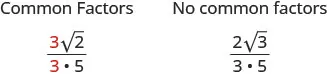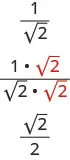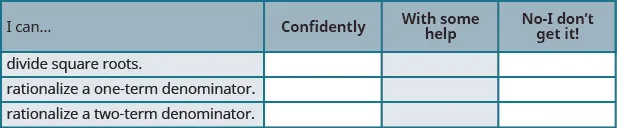Elementary Algebra 2e

# 9.5Divide Square Roots

Elementary Algebra 2e9.5 Divide Square Roots

### Learning Objectives

By the end of this section, you will be able to:

• Divide square roots
• Rationalize a one-term denominator
• Rationalize a two-term denominator

### Be Prepared 9.12

Before you get started, take this readiness quiz.

Find a fraction equivalent to $5858$ with denominator 48.
If you missed this problem, review Example 1.64.

### Be Prepared 9.13

Simplify: $(5)2(5)2$.
If you missed this problem, review Example 9.48.

### Be Prepared 9.14

Multiply: $(7+3x)(7−3x)(7+3x)(7−3x)$.
If you missed this problem, review Example 6.54.

### Divide Square Roots

We know that we simplify fractions by removing factors common to the numerator and the denominator. When we have a fraction with a square root in the numerator, we first simplify the square root. Then we can look for common factors.### Example 9.60

Simplify: $546546$.

### Try It 9.119

Simplify: $328328$.

### Try It 9.120

Simplify: $75157515$.

### Example 9.61

Simplify: $6−24126−2412$.

### Try It 9.121

Simplify: $8−40108−4010$.

### Try It 9.122

Simplify: $10−752010−7520$.

We have used the Quotient Property of Square Roots to simplify square roots of fractions. The Quotient Property of Square Roots says

$ab=ab,b≠0ab=ab,b≠0$

Sometimes we will need to use the Quotient Property of Square Roots ‘in reverse’ to simplify a fraction with square roots.

$ab=ab,b≠0ab=ab,b≠0$

We will rewrite the Quotient Property of Square Roots so we see both ways together. Remember: we assume all variables are greater than or equal to zero so that their square roots are real numbers.

### Quotient Property of Square Roots

If a, b are non-negative real numbers and $b≠0b≠0$, then

$ab=abandab=abab=abandab=ab$

We will use the Quotient Property of Square Roots ‘in reverse’ when the fraction we start with is the quotient of two square roots, and neither radicand is a perfect square. When we write the fraction in a single square root, we may find common factors in the numerator and denominator.

### Example 9.62

Simplify: $27752775$.

### Try It 9.123

Simplify: $4810848108$.

### Try It 9.124

Simplify: $96549654$.

We will use the Quotient Property for Exponents, $aman=am−naman=am−n$, when we have variables with exponents in the radicands.

### Example 9.63

Simplify: $6y52y6y52y$.

### Try It 9.125

Simplify: $12r36r12r36r$.

### Try It 9.126

Simplify: $14p92p514p92p5$.

### Example 9.64

Simplify: $72x3162x72x3162x$.

### Try It 9.127

Simplify: $50s3128s50s3128s$.

### Try It 9.128

Simplify: $75q5108q75q5108q$.

### Example 9.65

Simplify: $147ab83a3b4147ab83a3b4$.

### Try It 9.129

Simplify: $162x10y22x6y6162x10y22x6y6$.

### Try It 9.130

Simplify: $300m3n73m5n300m3n73m5n$.

### Rationalize a One Term Denominator

Before the calculator became a tool of everyday life, tables of square roots were used to find approximate values of square roots. Figure 9.3 shows a portion of a table of squares and square roots. Square roots are approximated to five decimal places in this table.

Figure 9.3 A table of square roots was used to find approximate values of square roots before there were calculators.

If someone needed to approximate a fraction with a square root in the denominator, it meant doing long division with a five decimal-place divisor. This was a very cumbersome process.

For this reason, a process called rationalizing the denominator was developed. A fraction with a radical in the denominator is converted to an equivalent fraction whose denominator is an integer. This process is still used today and is useful in other areas of mathematics, too.

### Rationalizing the Denominator

The process of converting a fraction with a radical in the denominator to an equivalent fraction whose denominator is an integer is called rationalizing the denominator.

Square roots of numbers that are not perfect squares are irrational numbers. When we rationalize the denominator, we write an equivalent fraction with a rational number in the denominator.

Let’s look at a numerical example.

$Suppose we need an approximate value for the fraction.12A five decimal place approximation to2is1.41421.11.41421Without a calculator, would you want to do this division?1.414211.0Suppose we need an approximate value for the fraction.12A five decimal place approximation to2is1.41421.11.41421Without a calculator, would you want to do this division?1.414211.0$

But we can find a fraction equivalent to $1212$ by multiplying the numerator and denominator by $22$.Now if we need an approximate value, we divide $21.4142121.41421$. This is much easier.

Even though we have calculators available nearly everywhere, a fraction with a radical in the denominator still must be rationalized. It is not considered simplified if the denominator contains a square root.

Similarly, a square root is not considered simplified if the radicand contains a fraction.

### Simplified Square Roots

A square root is considered simplified if there are

• no perfect-square factors in the radicand
• no fractions in the radicand
• no square roots in the denominator of a fraction

To rationalize a denominator, we use the property that $(a)2=a(a)2=a$. If we square an irrational square root, we get a rational number.

We will use this property to rationalize the denominator in the next example.

### Example 9.66

Simplify: $4343$.

### Try It 9.131

Simplify: $5353$.

### Try It 9.132

Simplify: $6565$.

### Example 9.67

Simplify: $−836−836$.

### Try It 9.133

Simplify: $525525$.

### Try It 9.134

Simplify: $−943−943$.

Always simplify the radical in the denominator first, before you rationalize it. This way the numbers stay smaller and easier to work with.

### Example 9.68

Simplify: $512512$.

### Try It 9.135

Simplify: $718718$.

### Try It 9.136

Simplify: $332332$.

### Example 9.69

Simplify: $11281128$.

### Try It 9.137

Simplify: $327327$.

### Try It 9.138

Simplify: $10501050$.

### Rationalize a Two-Term Denominator

When the denominator of a fraction is a sum or difference with square roots, we use the Product of Conjugates pattern to rationalize the denominator.

$(a−b)(a+b)(2−5)(2+5)a2−b222−(5)24−5−1(a−b)(a+b)(2−5)(2+5)a2−b222−(5)24−5−1$

When we multiply a binomial that includes a square root by its conjugate, the product has no square roots.

### Example 9.70

Simplify: $44+244+2$.

### Try It 9.139

Simplify: $22+322+3$.

### Try It 9.140

Simplify: $55+355+3$.

### Example 9.71

Simplify: $52−352−3$.

### Try It 9.141

Simplify: $31−531−5$.

### Try It 9.142

Simplify: $24−624−6$.

### Example 9.72

Simplify: $3u−63u−6$.

### Try It 9.143

Simplify: $5x+25x+2$.

### Try It 9.144

Simplify: $10y−310y−3$.

### Example 9.73

Simplify: $x+7x−7x+7x−7$.

### Try It 9.145

Simplify: $p+2p−2p+2p−2$.

### Try It 9.146

Simplify: $q−10q+10q−10q+10$.

### Media

Access this online resource for additional instruction and practice with dividing and rationalizing.

### Section 9.5 Exercises

#### Practice Makes Perfect

Divide Square Roots

In the following exercises, simplify.

317.

$27 6 27 6$

318.

$50 10 50 10$

319.

$72 9 72 9$

320.

$243 6 243 6$

321.

$2 − 32 8 2 − 32 8$

322.

$3 + 27 9 3 + 27 9$

323.

$6 + 45 6 6 + 45 6$

324.

$10 − 200 20 10 − 200 20$

325.

$80 125 80 125$

326.

$72 200 72 200$

327.

$128 72 128 72$

328.

$48 75 48 75$

329.

$8x62x28x62x2$ $200m598m200m598m$

330.

$10y35y10y35y$ $108n7243n3108n7243n3$

331.

$75 r 3 108 r 75 r 3 108 r$

332.

$196 q 5 484 q 196 q 5 484 q$

333.

$108 p 5 q 2 3 p 3 q 6 108 p 5 q 2 3 p 3 q 6$

334.

$98 r s 10 2 r 3 s 4 98 r s 10 2 r 3 s 4$

335.

$320 m n 5 45 m 7 n 3 320 m n 5 45 m 7 n 3$

336.

$810 c 3 d 7 1000 c 5 d 810 c 3 d 7 1000 c 5 d$

337.

$98 14 98 14$

338.

$72 18 72 18$

339.

$5 + 125 15 5 + 125 15$

340.

$6 − 45 12 6 − 45 12$

341.

$96 150 96 150$

342.

$28 63 28 63$

343.

$26 y 7 2 y 26 y 7 2 y$

344.

$15 x 3 3 x 15 x 3 3 x$

Rationalize a One-Term Denominator

In the following exercises, simplify and rationalize the denominator.

345.

$10 6 10 6$

346.

$8 3 8 3$

347.

$6 7 6 7$

348.

$4 5 4 5$

349.

$3 13 3 13$

350.

$10 11 10 11$

351.

$10 3 10 10 3 10$

352.

$2 5 2 2 5 2$

353.

$4 9 5 4 9 5$

354.

$9 2 7 9 2 7$

355.

$− 9 2 3 − 9 2 3$

356.

$− 8 3 6 − 8 3 6$

357.

$3 20 3 20$

358.

$4 27 4 27$

359.

$7 40 7 40$

360.

$8 45 8 45$

361.

$19 175 19 175$

362.

$17 192 17 192$

Rationalize a Two-Term Denominator

In the following exercises, simplify by rationalizing the denominator.

363.

$33+1133+11$ $81−581−5$

364.

$44+744+7$ $72−672−6$

365.

$55+655+6$ $63−763−7$

366.

$66+566+5$ $54−1154−11$

367.

$3 m − 5 3 m − 5$

368.

$5 n − 7 5 n − 7$

369.

$2 x − 6 2 x − 6$

370.

$7 y + 3 7 y + 3$

371.

$r + 5 r − 5 r + 5 r − 5$

372.

$s − 6 s + 6 s − 6 s + 6$

373.

$150 x 2 y 6 6 x 4 y 2 150 x 2 y 6 6 x 4 y 2$

374.

$80 p 3 q 5 p q 5 80 p 3 q 5 p q 5$

375.

$15 5 15 5$

376.

$3 5 8 3 5 8$

377.

$8 54 8 54$

378.

$12 20 12 20$

379.

$3 5 + 5 3 5 + 5$

380.

$20 4 − 3 20 4 − 3$

381.

$2 x − 3 2 x − 3$

382.

$5 y − 7 5 y − 7$

383.

$x + 8 x − 8 x + 8 x − 8$

384.

$m − 3 m + 3 m − 3 m + 3$

#### Everyday Math

385.

A supply kit is dropped from an airplane flying at an altitude of 250 feet. Simplify $2501625016$ to determine how many seconds it takes for the supply kit to reach the ground.

386.

A flare is dropped into the ocean from an airplane flying at an altitude of 1,200 feet. Simplify $120016120016$ to determine how many seconds it takes for the flare to reach the ocean.

#### Writing Exercises

387.
1. Simplify $273273$ and explain all your steps.
2. Simplify $275275$ and explain all your steps.
3. Why are the two methods of simplifying square roots different?
388.
1. Approximate $1212$ by dividing $11.41411.414$ using long division without a calculator.
2. Rationalizing the denominator of $1212$ gives $22.22.$ Approximate $2222$ by dividing $1.41421.4142$ using long division without a calculator.
3. Do you agree that rationalizing the denominator makes calculations easier? Why or why not?

#### Self Check

After completing the exercises, use this checklist to evaluate your mastery of the objectives of this section.After looking at the checklist, do you think you are well-prepared for the next section? Why or why not?

Order a print copy

As an Amazon Associate we earn from qualifying purchases.